# David number

Jana and David train the addition of the decimal numbers so that each of them will write a single number and these two numbers then add up. The last example was 11.11. David's number had the same number of digits before the decimal point, the Jane's number also. David's number was written in different numbers, Jane's number had exactly two digits the same. Find the largest possible number David could write.

Correct result:

D =  0.9

#### Solution:

$D=0.9=\frac{9}{10}$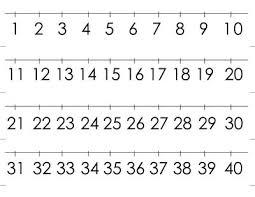We would be pleased if you find an error in the word problem, spelling mistakes, or inaccuracies and send it to us. Thank you!## Next similar math problems:

• Number train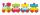The numbers 1,2,3,4,5,6,7,8 and 9 traveled by train. The train had three cars and each was carrying just three numbers. No. 1 rode in the first carriage, and in the last carriage was all odd numbers. The conductor calculated sum of the numbers in the firs
• Flowers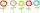The flower has six flowers, and each flower has a number. These are the numbers: 20,40,39,28,8,9. What number will be in the middle of the flower so that the numbers come from the flowers when we subtract and add?
• DecideThe rectangle is divided into seven fields. On each box is to write just one of the numbers 1, 2 and 3. Mirek argue that it can be done so that the sum of the two numbers written next to each other was always different. Zuzana (Susan) instead argue that i
• The largest numberFind the largest integer such that: 1. No figures is not repeat, 2. multiplication of every two digits is odd, 3. addition all digits is odd.
• Alarm clockThe old watchmaker has a unique digital alarm in its collection that rings whenever the sum of digits of the alarm is equal to 21. Find out when the alarm clock will ring. What is their number? List all options . ..
• Unknown numberSamuel wrote unknown number. Then he had add 200000 to the number and the result multiply by three. When it calculated he was surprised, because the result would have received anyway, if write digit to the end of original number. Find unknown number.
• Star equationWrite digits instead of stars so that the sum of the written digits is odd and is true equality: 42 · ∗8 = 2 ∗∗∗
• Digits of age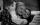The product of the digits of Andrew age as 6 years ago and not equal to 0. Andrew age is also the smallest possible age with this two conditions. After how many years will be the product of the digits of Andrew age again the same as today?
• By sixFrom the digits 1,2,3,4 we create the long integer number 123412341234. .. .. , which will have 962 digits. Is this number divisible by 6?
• Sports studentsThere are 120 athletes, 48 volleyball players, and 72 handball players at the school with extended sports training. Is it possible to divide sports students into groups so that the number in each group is the same and expressed by the largest possible num
• Twenty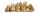Twenty rabbits are put in 4 cells so that there are different number of rabbits in each cell contains at least 3 rabbits. What is the largest possible number of rabbits in one cell
• Mouse Hryzka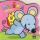Mouse Hryzka found 27 identical cubes of cheese. She first put in a large cube out of them and then waited for a while before the cheese cubes stuck together. Then from every wall of the big cube she will eats the middle cube. Then she also eats the cube
• Three friendsThree friends had balls in ratio 2: 7: 4 at the start of the game. Could they have the same number of balls at the end of the game? Write 0, if not, or write the minimum number of balls they had together.
• TilesThe room has dimensions 12 m and 5.6 m. Determine the number of square tiles and their largest possible size to cover them room's floor.
• Whole numbersPavol wrote down a number that is both rational and a whole number. What is one possible number she could have written down?
• Bus vs. trainThe bus started from point A 10 minutes before the train started from the same place. The bus went an average speed of 49 km/h, train 77 km/h. To point B train and bus arrived simultaneously. Calculate the time of the train journey, if train and bus trave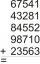Fill letters instead of digits so the indicated sum (equal letters represent equal digits). What number is hidden under the letter J? A A H A H O A H O J -------------------------- 4 3 2 1